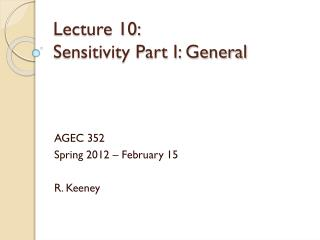# Lecture 10: Sensitivity Part I: General - PowerPoint PPT PresentationDownload PresentationLecture 10: Sensitivity Part I: General

Lecture 10: Sensitivity Part I: General
Download Presentation## Lecture 10: Sensitivity Part I: General

- - - - - - - - - - - - - - - - - - - - - - - - - - - E N D - - - - - - - - - - - - - - - - - - - - - - - - - - -
##### Presentation Transcript

1. Lecture 10: Sensitivity Part I: General AGEC 352 Spring 2012 – February 15 R. Keeney

2. Simple Problem Corner Points: (x,y) (10,0) (10,10) (0,10) Solution x = 10, y = 10, Z=20 Both constraints bind

3. Two Initial Questions • 1) If we add a constraint, is the decision maker in the problem… • Better off? Worse off? Indifferent? • 2) If we remove a constraint, is the decision maker in the problem… • Better off? Worse off? Indifferent? • Try to find examples that produce each result to prove to ourselves the implications

4. Question 1: A new constraint with no impact on the objective variable • The new constraint says the sum of x and y has to be less than 100 • The maximized value from the original problem was Z=x+y=20 • Clearly the objective variable will be unaffected by this new constraint • DM is indifferent to this addition

5. Question 1: A new constraint with an adverse impact on the objective • Is our solution to the initial problem still feasible? • 2(10)+10 = 30 which fails the new constraint

6. Question 1: A new constraint generating an improvement • Added constraints can have two impacts on the feasible space • Leave it unchanged • Shrink it • Since the new constraint cannot expand the feasible space it would be impossible to find a new constraint that makes the decision maker better off.

7. Question 2: Removing a constraint • Left for you to think through • Key concept: • Adding a constraint can leave the feasible space unchanged or shrink it • Show that removal of a constraint can leave the feasible space unchanged or expand it • Implications for the decision maker’s objective variable are going to be opposite

8. Simple 3 variable problem q is a new choice variable q competes with x in the constraint q is worth ½ as much as x in the objective equation Note that y is unaffected by the new choice variable q. Increasing y increases the objective variable so we can set y to 10 (its highest level) and just consider the choice between x and q.

9. 2 Initial Questions when adding a choice variable • Will the addition of a choice variable make the decision maker… • Better off? Worse off? Indifferent? • Will the removal of a choice variable make the decision maker… • Better off? Worse off? Indifferent? • Again work with a simple example to try and prove which are possible.

10. Impact of Adding q to the problem • Optimal y = 10 is assumed but not shown • Choice between x and q graphed • Objective says use only x and set q=0 • Adding q to the problem has no impact in this instance

11. Another possibility of adding q • Now q enters the objective equation with a coefficient of 1.5 • Graphical solution says x=0,q=10 • Remember y=10 • New Z = 10 + (1.5)(10) • So adding q increased the objective value

12. Can a new decision variable be deterimental? • Not possible unless it comes with a constraint that forces its value to be a non-zero value • In this example, q was initially not worth pursuing, so the solution was to just set it to zero • What about removing a decision variable? • Beginning with the problem having x,y,qconsider the impact of taking q away (returning to the initial problem) • What possibilities exist for the decision maker being better/worse/indifferent?

13. Review Exercises • The minimization case is left to you in the handout to work out the sensitivity of the objective variable to added constraints or decision variable • Look through the review handout and ask any questions on Wednesday that you do not understand • Wednesday we will review shadow price sensitivity

14. General Sensitivity Summary • Adding a constraint • Worse off • Z decreases for max • Z increases for min • Indifferent (Z is the same) • Adding a variable • Better off • Z increases for max • Z decreases for min • Indifferent (Z is the same)